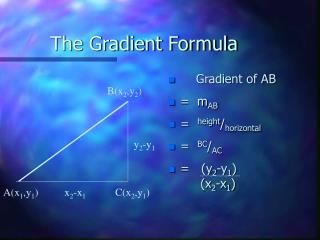Download Presentation# The Gradient Formula - PowerPoint PPT PresentationDownload Presentation- - - - - - - - - - - - - - - - - - - - - - - - - - - E N D - - - - - - - - - - - - - - - - - - - - - - - - - - -
##### Presentation Transcript

1. The Gradient Formula • Gradient of AB • = mAB • = height/horizontal • = BC/AC • = (y2-y1) (x2-x1) B(x2,y2) y2-y1 A(x1,y1) x2-x1 C(x2,y1)

2. The Gradient Formula ctd. • Summary • If A is (x1,y1) and B is (x2,y2) • then the gradient of AB is given by • mAB = (y2-y1) (x2-x1)

3. Example 4 P is (5, 9) and Q is (7,17). • mPQ = (y2-y1) (x2-x1) • = (17 - 9) • ( 7 - 5) • = 8/2 • = 4 NB: line looks like Q P

4. Example 5 E is (15, 9) and F is (7,17). • mEF = (y2-y1) (x2-x1) • = (17 - 9) • ( 7 - 15) • = 8/-8 • = -1 NB: line looks like F E

5. Example 6 V is (-5, -9) and W is (12,-9). • mVW = (y2-y1) (x2-x1) • = (-9 -(- 9)) • ( 12 - (-5)) • = 0/17 • = 0 • NB: A horizontal line has no steepness. NB: line looks like V W

6. Example 7 S is (5, -9) and T is (5, 12). • mST = (y2-y1) (x2-x1) • = (12 -(- 9)) • ( 5 - 5) • = 21/0 • and this is undefined • or infinite NB: line looks like T Vertical line has infinite gradient. S